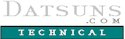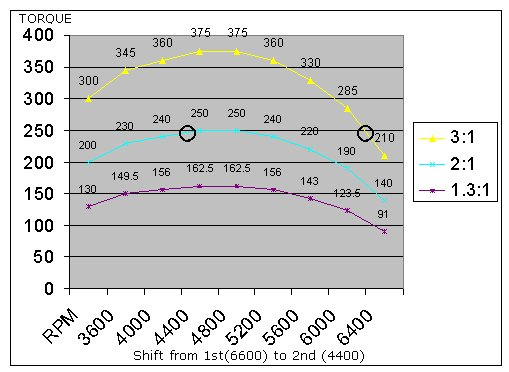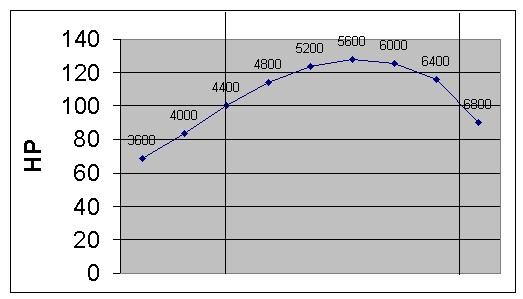DATSUNS home

Technical Dept Home

SOFTWARE

Calculate your
shift point

(Excel)

Optimal shift points
By Dave Lum - 10/22/98

Looking to get maximum effect out of your car when accelerating? Try optimizing the RPM's you shift your car at. If you're shifting at redline, you're probably not getting the best acceleration out of your car - in fact, the optimum shift RPM will probably be different for each gear. You can calculate the optimum shift points, but it takes two pieces of information : Gear ratios of all your gears, and a chart of your torque curve.

If you don't understand torque curves, you may want to take a look at the horsepower article in my Technical Department before reading any farther.

The trick here is to keep your engine in the meaty part of the torque curve, as your acceleration curve will match your torque curve - meaning if your torque peak is at 3000rpm, that's the RPM your car accelerates the hardest. How do you keep the engine in the best part of the curve? Shift when you will be at an RPM where the car will make more torque AFTER you shift than in your current gear. This may sound confusing, but I will give some examples to help demonstrate this. Gear ratio is important because you need to know what your RPM's will drop to once you shift. EXAMPLE : If you're at 6500rpm in first, you need the gear ratio to know what RPM that will translates to once you shift to 2nd.

Below is a torque curve for a fictitious engine :

 Engine Torque Gear ratio Now with your gear ratio in hand, figure out how many RPM's will drop going from 1st to 2nd. In this case, it'll drop by 1/3 (2:1 divided by 3:1). So shifting from 1st to 2nd at 6000rpm, it will drop to 4000rpm. This means you're going from 330ft/lbs (see chart - 6000rpm in 1st) to 220ft/lbs (6000rpm * .6666) = 4000rpm in 2nd). Not what you want - you want these numbers to be the same. How about 6400? 285 vs 240 - much better, but let's try 6800 : 210 vs about 250. Oops, too far. In this case, optimum shift point is between 6400 and 6800rpm for the 1-2 shift.It works the same for each consecutive gear, as the shift RPM may be different for each gear. Below are graphs representing  how it looks on "paper". 3:1 2:1 1.3:1 3600 100 300 200 130 4000 110 330 220 143 4400 120 360 240 156 4800 125 375 250 163 5200 125 375 250 163 5600 120 360 240 156 6000 110 330 220 143 6400 95 285 190 124 6800 70 210 140 91 Gear --> 1st 2nd 3rd

Notice anything? The two torque outputs are almost the same (6600 in first and 4400 in second), which is what you're going for to achieve maximum acceleration. What does it look like on the horsepower side? Take a look at the chart below :Well what do you know! You bracket the HP peak. HP after the shift is the same as HP before the shift. Now we know shifting at the torque peak isn't the answer, and neither is shifting at the HP peak.

The math required : next higher gear ratio / current gear ratio (i,e. second gear divided by first) = X

X * shiftrpm = nextrpm, where shiftrpm is the point your shifting AT, and nextrpm will be the RPM of the next gear at the same speed.

firstgeartorque = torque at shiftrpm times current gear ratio (example, at 6400rpm we have 95ft/lbs, multiply this by our gear ratio in 1st (3:1, or just 3) and you get about 245ft/lbs.

secondgeartorque = torque at nextrpm times next gear ratio (in out example, we have 120ft/lbs @ 4400rpm, times 2:1 (second gear ratio), and you get 240ft/lbs.

Change shiftrpm until firstgeartorque is equal (or close to) secondgeartorque.